Home Practice
For learners and parents For teachers and schools
Textbooks
Full catalogue
Pricing SupportLog in

We think you are located in United States. Is this correct?

# 5.6 The cosine function

## 5.6 The cosine function (EMBH3)

### Revision (EMBH4)

#### Functions of the form $$y = \cos \theta$$ for $$\text{0}\text{°} \leq \theta \leq \text{360}\text{°}$$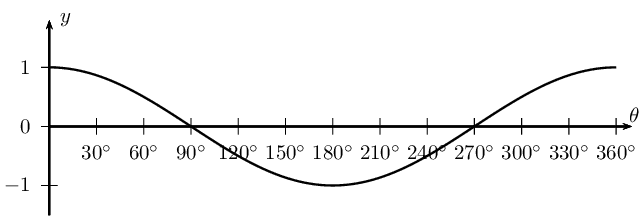• The period is $$\text{360}\text{°}$$ and the amplitude is $$\text{1}$$.

• Domain: $$[\text{0}\text{°};\text{360}\text{°}]$$

For $$y = \cos \theta$$, the domain is $$\{ \theta: \theta \in \mathbb{R} \}$$, however in this case, the domain has been restricted to the interval $$\text{0}\text{°} \leq \theta \leq \text{360}\text{°}$$.

• Range: $$\left[-1;1\right]$$

• $$x$$-intercepts: $$\left(\text{90}\text{°};0\right)$$, $$\left(\text{270}\text{°};0\right)$$

• $$y$$-intercept: $$\left(\text{0}\text{°};1\right)$$

• Maximum turning points: $$\left(\text{0}\text{°};1\right)$$, $$\left(\text{360}\text{°};1\right)$$

• Minimum turning point: $$\left(\text{180}\text{°};-1\right)$$

#### Functions of the form $$y = a \cos \theta + q$$

Cosine functions of the general form $$y = a \cos \theta + q$$, where $$a$$ and $$q$$ are constants.

The effects of $$a$$ and $$q$$ on $$f(\theta) = a \cos \theta + q$$:

• The effect of $$q$$ on vertical shift

• For $$q>0$$, $$f(\theta)$$ is shifted vertically upwards by $$q$$ units.

• For $$q<0$$, $$f(\theta)$$ is shifted vertically downwards by $$q$$ units.

• The effect of $$a$$ on shape

• For $$a>1$$, the amplitude of $$f(\theta)$$ increases.

• For $$0<a<1$$, the amplitude of $$f(\theta)$$ decreases.

• For $$a<0$$, there is a reflection about the $$x$$-axis.

• For $$-1 < a < 0$$, there is a reflection about the $$x$$-axis and the amplitude decreases.

• For $$a < -1$$, there is a reflection about the $$x$$-axis and the amplitude increases.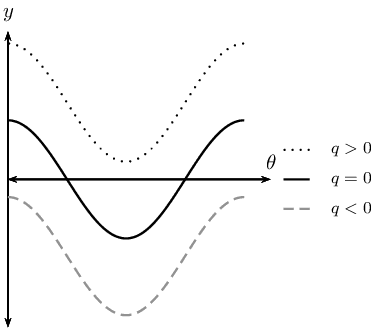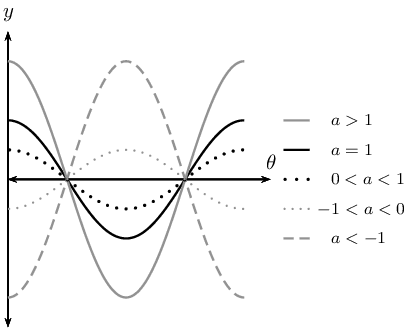temp text

## Revision

Textbook Exercise 5.24

On separate axes, accurately draw each of the following functions for $$\text{0}\text{°} \leq \theta \leq \text{360}\text{°}$$:

• Use tables of values if necessary.
• Use graph paper if available.

For each function in the previous problem determine the following:

• Period
• Amplitude
• Domain and range
• $$x$$- and $$y$$-intercepts
• Maximum and minimum turning points

$$y_1 = \cos \theta$$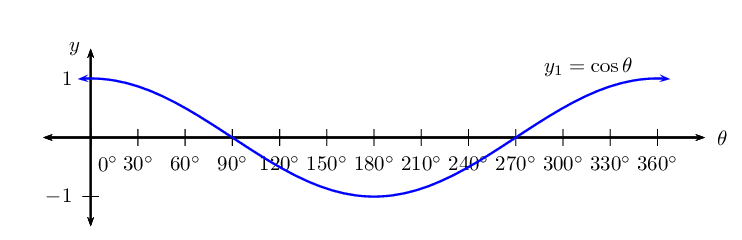$$y_2 = - 3 \cos \theta$$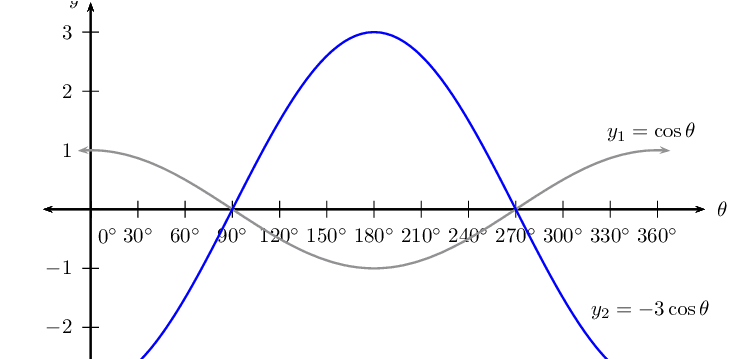$$y_3 = \cos \theta + 2$$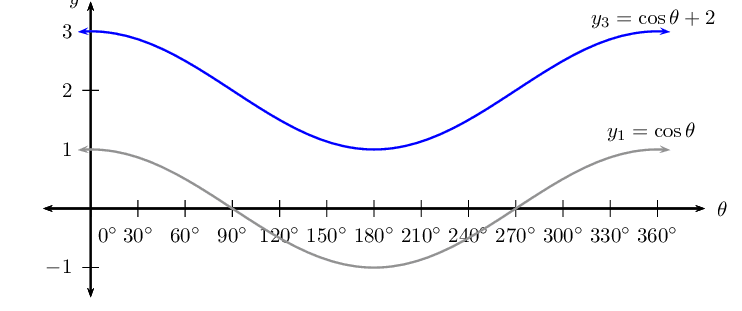$$y_4 = \frac{1}{2} \cos \theta - 1$$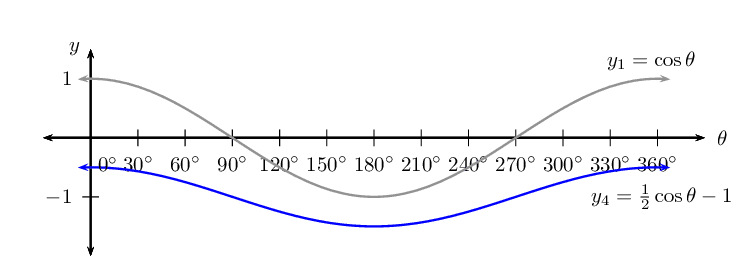### Functions of the form $$y=\cos (k\theta)$$ (EMBH5)

We now consider cosine functions of the form $$y = \cos k\theta$$ and the effects of parameter $$k$$.

## The effects of $$k$$ on a cosine graph

1. Complete the following table for $$y_1 = \cos \theta$$ for $$-\text{360}\text{°} \leq \theta \leq \text{360}\text{°}$$:
 θ $$-\text{360}$$$$\text{°}$$ $$-\text{300}$$$$\text{°}$$ $$-\text{240}$$$$\text{°}$$ $$-\text{180}$$$$\text{°}$$ $$-\text{120}$$$$\text{°}$$ $$-\text{60}$$$$\text{°}$$ $$\text{0}$$$$\text{°}$$ $$\cos \theta$$ θ $$\text{60}$$$$\text{°}$$ $$\text{120}$$$$\text{°}$$ $$\text{180}$$$$\text{°}$$ $$\text{240}$$$$\text{°}$$ $$\text{300}$$$$\text{°}$$ $$\text{360}$$$$\text{°}$$ $$\cos \theta$$
2. Use the table of values to plot the graph of $$y_1 = \cos \theta$$ for $$-\text{360}\text{°} \leq \theta \leq \text{360}\text{°}$$.

3. On the same system of axes, plot the following graphs:

1. $$y_2 = \cos (-\theta)$$
2. $$y_3 = \cos 3\theta$$
3. $$y_4 = \cos \frac{3\theta}{4}$$
4. Use your sketches of the functions above to complete the following table:

 $$y_1$$ $$y_2$$ $$y_3$$ $$y_4$$ period amplitude domain range maximum turning points minimum turning points $$y$$-intercept(s) $$x$$-intercept(s) effect of $$k$$
5. What do you notice about $$y_1 = \cos \theta$$ and $$y_2 = \cos (-\theta)$$?

6. Is $$\cos (-\theta) = -\cos \theta$$ a true statement? Explain your answer.

7. Can you deduce a formula for determining the period of $$y = \cos k\theta$$?

The effect of the parameter $$k$$ on $$y = \cos k\theta$$

The value of $$k$$ affects the period of the cosine function.

• For $$k > 0$$:

For $$k > 1$$, the period of the cosine function decreases.

For $$0 < k < 1$$, the period of the cosine function increases.

• For $$k < 0$$:

For $$-1 < k < 0$$, the period increases.

For $$k < -1$$, the period decreases.

Negative angles: $\cos (-\theta) = \cos \theta$ Notice that for negative values of $$\theta$$, the graph is not reflected about the $$x$$-axis.

Calculating the period:

To determine the period of $$y = \cos k\theta$$ we use, $\text{Period } = \frac{\text{360}\text{°}}{|k|}$ where $$|k|$$ is the absolute value of $$k$$.

 $$0 < k < 1$$ $$-1 < k < 0$$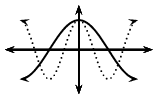$$k > 1$$ $$k < -1$$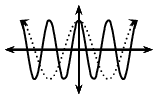## Worked example 22: Cosine function

1. Sketch the following functions on the same set of axes for $$-\text{180}\text{°} \leq \theta \leq \text{180}\text{°}$$.
1. $$y_1 = \cos \theta$$
2. $$y_2 = \cos \frac{\theta}{2}$$
2. For each function determine the following:

1. Period
2. Amplitude
3. Domain and range
4. $$x$$- and $$y$$-intercepts
5. Maximum and minimum turning points

### Examine the equations of the form $$y = \cos k\theta$$

Notice that for $$y_2 = \cos \frac{\theta}{2}$$, $$k < 1$$ therefore the period of the graph increases.

### Complete a table of values

 θ $$-\text{180}$$$$\text{°}$$ $$-\text{135}$$$$\text{°}$$ $$-\text{90}$$$$\text{°}$$ $$-\text{45}$$$$\text{°}$$ $$\text{0}$$$$\text{°}$$ $$\text{45}$$$$\text{°}$$ $$\text{90}$$$$\text{°}$$ $$\text{135}$$$$\text{°}$$ $$\text{180}$$$$\text{°}$$ $$\cos \theta$$ $$-\text{1}$$ $$-\text{0,71}$$ $$\text{0}$$ $$\text{0,71}$$ $$\text{1}$$ $$\text{0,71}$$ $$\text{0}$$ $$-\text{0,71}$$ $$-\text{1}$$ $$\cos \frac{\theta}{2}$$ $$\text{0}$$ $$\text{0,38}$$ $$\text{0,71}$$ $$\text{0,92}$$ $$\text{1}$$ $$\text{0,92}$$ $$\text{0,71}$$ $$\text{0,38}$$ $$\text{0}$$

### Sketch the cosine graphs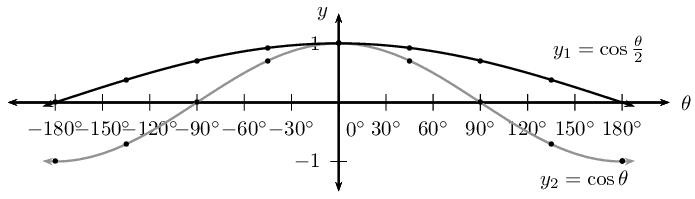### Complete the table

 $$y_1 = \cos \theta$$ $$y_2 = \cos \frac{\theta}{2}$$ period $$\text{360}\text{°}$$ $$\text{720}\text{°}$$ amplitude $$\text{1}$$ $$\text{1}$$ domain $$[-\text{180}\text{°};\text{180}\text{°}]$$ $$[-\text{180}\text{°};\text{180}\text{°}]$$ range $$[-1;1]$$ $$[0;1]$$ maximum turning points $$(\text{0}\text{°};1)$$ $$(\text{0}\text{°};1)$$ minimum turning points $$(-\text{180}\text{°};-1) \text{ and } (\text{180}\text{°};-1)$$ none $$y$$-intercept(s) $$(\text{0}\text{°};1)$$ $$(\text{0}\text{°};1)$$ $$x$$-intercept(s) $$(-\text{90}\text{°};0) \text{ and } (\text{90}\text{°};0)$$ $$(-\text{180}\text{°};0) \text{ and } (\text{180}\text{°};0)$$

#### Discovering the characteristics

For functions of the general form: $$f(\theta) = y =\cos k\theta$$:

Domain and range

The domain is $$\{ \theta: \theta \in \mathbb{R} \}$$ because there is no value for $$\theta$$ for which $$f(\theta)$$ is undefined.

The range is $$\{ f(\theta): -1 \leq f(\theta) \leq 1, f(\theta) \in \mathbb{R} \}$$ or $$[-1;1]$$.

Intercepts

The $$x$$-intercepts are determined by letting $$f(\theta) = 0$$ and solving for $$\theta$$.

The $$y$$-intercept is calculated by letting $$\theta = \text{0}\text{°}$$ and solving for $$f(\theta)$$. \begin{align*} y &= \cos k\theta \\ &= \cos \text{0}\text{°} \\ &= 1 \end{align*} This gives the point $$(\text{0}\text{°};1)$$.

temp text

## Cosine functions of the form $$y = \cos k\theta$$

Textbook Exercise 5.25

Sketch the following functions for $$-\text{180}\text{°} \leq \theta \leq \text{180}\text{°}$$. For each graph determine:

• Period
• Amplitude
• Domain and range
• $$x$$- and $$y$$-intercepts
• Maximum and minimum turning points

$$f(\theta) =\cos 2\theta$$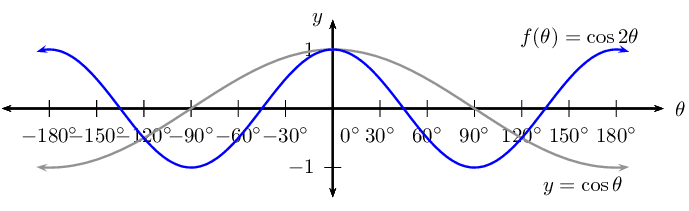For $$f(\theta) =\cos 2 \theta$$:

\begin{align*} \text{Period: } & \text{180}\text{°} \\ \text{Amplitude: } & 1 \\ \text{Domain: } & [-\text{180}\text{°};\text{180}\text{°}] \\ \text{Range: } & [-1;1] \\ x\text{-intercepts: } & (-\text{135}\text{°};0); (-\text{45}\text{°};0); (\text{45}\text{°};0); (\text{135}\text{°};0) \\ y\text{-intercepts: } & (\text{0}\text{°};1) \\ \text{Max. turning point: } & (-\text{180}\text{°};1); (\text{0}\text{°};1); (\text{180}\text{°};1) \\ \text{Min. turning point: } & (-\text{90}\text{°};-1); (\text{90}\text{°};-1) \end{align*}

$$g(\theta) =\cos \frac{\theta}{3}$$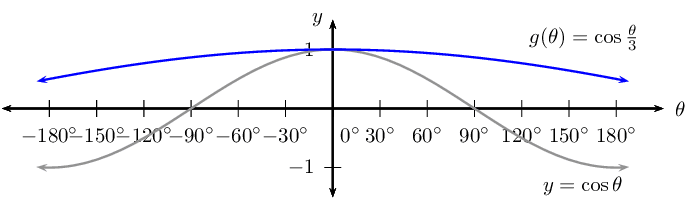For $$g(\theta) =\cos \frac{\theta}{3}$$:

\begin{align*} \text{Period: } & \text{1 080}\text{°} \\ \text{Amplitude: } & 1 \\ \text{Domain: } & [-\text{180}\text{°};\text{180}\text{°}] \\ \text{Range: } & [\frac{1}{2};1] \\ x\text{-intercepts: } & \text{ none } \\ \text{Max. turning point: } & (\text{0}\text{°};1) \\ \text{Min. turning point: } & \text{ none } \end{align*}

$$h(\theta) =\cos (-2\theta)$$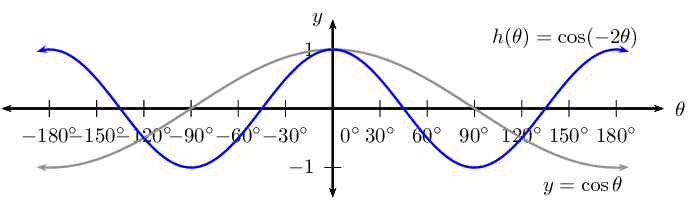For $$h(\theta) =\cos (-2\theta)$$:

\begin{align*} \text{Period: } & \text{180}\text{°} \\ \text{Amplitude: } & 1 \\ \text{Domain: } & [-\text{180}\text{°};\text{180}\text{°}] \\ \text{Range: } & [-1;1] \\ x\text{-intercepts: } & (-\text{135}\text{°};0); (-\text{45}\text{°};0); (\text{45}\text{°};0); (\text{135}\text{°};0) \\ y\text{-intercepts: } & (\text{0}\text{°};1) \\ \text{Max. turning point: } & (-\text{180}\text{°};1); (\text{0}\text{°};1); (\text{180}\text{°};1) \\ \text{Min. turning point: } & (-\text{90}\text{°};-1); (\text{90}\text{°};-1) \end{align*}

$$k(\theta) =\cos \frac{3\theta}{4}$$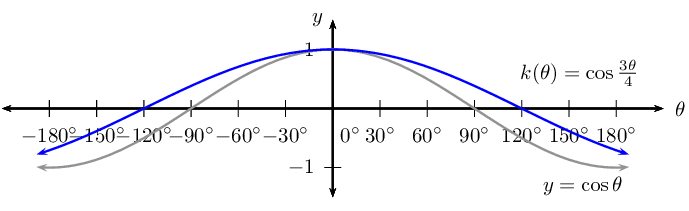For $$k(\theta) =\cos \frac{3\theta}{4}$$:

\begin{align*} \text{Period: } & \text{480}\text{°} \\ \text{Amplitude: } & 1 \\ \text{Domain: } & [-\text{180}\text{°};\text{180}\text{°}] \\ \text{Range: } & [-\frac{1}{\sqrt{2}};1] \\ x\text{-intercepts: } & (-\text{120}\text{°};0); (\text{120}\text{°};0) \\ y\text{-intercepts: } & (\text{0}\text{°};1) \\ \text{Max. turning point: } & (\text{0}\text{°};1) \\ \text{Min. turning point: } & \text{ none } \end{align*}

For each graph of the form $$f(\theta) =\cos k\theta$$, determine the value of $$k$$: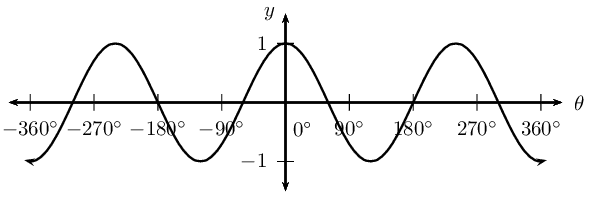\begin{align*} \text{Period } &= \frac{\text{720}\text{°}}{3 \text{ complete waves }} \\ &= \text{240}\text{°} \\ \therefore \frac{\text{360}\text{°}}{k} &= \text{240}\text{°} \\ \therefore k &= \frac{\text{360}\text{°}}{\text{240}\text{°} } \\ &= \frac{3}{2} \end{align*}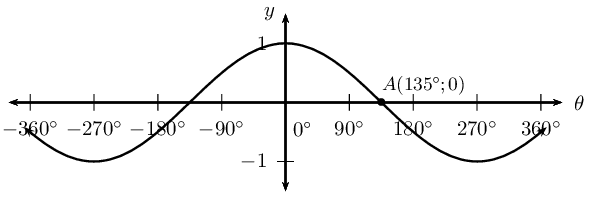\begin{align*} \text{For } y &= \cos \theta \\ 0 &= \cos \text{90}\text{°}\\ \text{So for } A(\text{135}\text{°};0) \qquad \text{90}\text{°} &= k \times \text{135}\text{°}\\ \therefore k &= \frac{\text{90}\text{°}}{\text{135}\text{°} } \\ &= \frac{2}{3} \end{align*}

### Functions of the form $$y=\cos\left(\theta +p\right)$$ (EMBH6)

We now consider cosine functions of the form $$y = \cos(\theta + p)$$ and the effects of parameter $$p$$.

## The effects of $$p$$ on a cosine graph

1. On the same system of axes, plot the following graphs for $$-\text{360}\text{°} \leq \theta \leq \text{360}\text{°}$$:

1. $$y_1 = \cos \theta$$
2. $$y_2 = \cos (\theta - \text{90}\text{°})$$
3. $$y_3 = \cos (\theta - \text{60}\text{°})$$
4. $$y_4 = \cos (\theta + \text{90}\text{°})$$
5. $$y_5 = \cos (\theta + \text{180}\text{°})$$
2. Use your sketches of the functions above to complete the following table:

 $$y_1$$ $$y_2$$ $$y_3$$ $$y_4$$ $$y_5$$ period amplitude domain range maximum turning points minimum turning points $$y$$-intercept(s) $$x$$-intercept(s) effect of $$p$$

The effect of the parameter on $$y = \cos(\theta + p)$$

The effect of $$p$$ on the cosine function is a horizontal shift (or phase shift); the entire graph slides to the left or to the right.

• For $$p > 0$$, the graph of the cosine function shifts to the left by $$p$$ degrees.

• For $$p < 0$$, the graph of the cosine function shifts to the right by $$p$$ degrees.

 $$p>0$$ $$p<0$$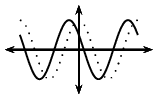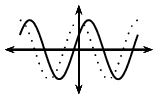## Worked example 23: Cosine function

1. Sketch the following functions on the same set of axes for $$-\text{360}\text{°} \leq \theta \leq \text{360}\text{°}$$.
1. $$y_1 = \cos \theta$$
2. $$y_2 = \cos (\theta + \text{30}\text{°})$$
2. For each function determine the following:

1. Period
2. Amplitude
3. Domain and range
4. $$x$$- and $$y$$-intercepts
5. Maximum and minimum turning points

### Examine the equations of the form $$y = \cos (\theta + p)$$

Notice that for $$y_1 = \cos \theta$$ we have $$p = 0$$ (no phase shift) and for $$y_2 = \cos (\theta + \text{30}\text{°})$$, $$p < 0$$ therefore the graph shifts to the left by $$\text{30}\text{°}$$.

### Complete a table of values

 θ $$-\text{360}$$$$\text{°}$$ $$-\text{270}$$$$\text{°}$$ $$-\text{180}$$$$\text{°}$$ $$-\text{90}$$$$\text{°}$$ $$\text{0}$$$$\text{°}$$ $$\text{90}$$$$\text{°}$$ $$\text{180}$$$$\text{°}$$ $$\text{270}$$$$\text{°}$$ $$\text{360}$$$$\text{°}$$ $$\cos \theta$$ $$\text{1}$$ $$\text{0}$$ $$-\text{1}$$ $$\text{0}$$ $$\text{1}$$ $$\text{0}$$ $$-\text{1}$$ $$\text{0}$$ $$\text{1}$$ $$\cos(\theta + \text{30}\text{°})$$ $$\text{0,87}$$ $$-\text{0,5}$$ $$-\text{0,87}$$ $$\text{0,5}$$ $$\text{0,87}$$ $$-\text{0,5}$$ $$-\text{0,87}$$ $$\text{0,5}$$ $$\text{0,87}$$

### Sketch the cosine graphs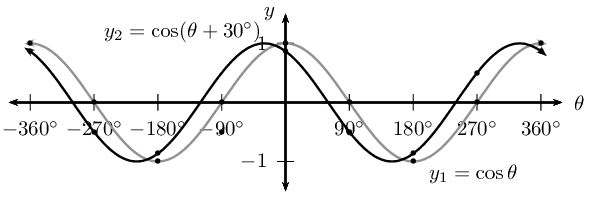### Complete the table

 $$y_1$$ $$y_2$$ period $$\text{360}\text{°}$$ $$\text{360}\text{°}$$ amplitude $$\text{1}$$ $$\text{1}$$ domain $$[-\text{360}\text{°};\text{360}\text{°}]$$ $$[-\text{360}\text{°};\text{360}\text{°}]$$ range $$[-1;1]$$ $$[-1;1]$$ maximum turning points $$(-\text{360}\text{°};1)$$, $$(\text{0}\text{°};1)$$ and $$(\text{360}\text{°};1)$$ $$(-\text{30}\text{°};1)$$ and $$(\text{330}\text{°};1)$$ minimum turning points $$(-\text{180}\text{°};-1)$$ and $$(\text{180}\text{°};-1)$$ $$(-\text{210}\text{°};-1)$$ and $$(\text{150}\text{°};-1)$$ $$y$$-intercept(s) $$(\text{0}\text{°};0)$$ $$(\text{0}\text{°};\text{0,87})$$ $$x$$-intercept(s) $$(-\text{270}\text{°};0)$$, $$(-\text{90}\text{°};0)$$, $$(\text{90}\text{°};0)$$ and $$(\text{270}\text{°};0)$$ $$(-\text{300}\text{°};0)$$, $$(-\text{120}\text{°};0)$$, $$(\text{60}\text{°};0)$$ and $$(\text{240}\text{°};0)$$

#### Discovering the characteristics

For functions of the general form: $$f(\theta) = y =\cos (\theta + p)$$:

Domain and range

The domain is $$\{ \theta: \theta \in \mathbb{R} \}$$ because there is no value for $$\theta$$ for which $$f(\theta)$$ is undefined.

The range is $$\{ f(\theta): -1 \leq f(\theta) \leq 1, f(\theta) \in \mathbb{R} \}$$.

Intercepts

The $$x$$-intercepts are determined by letting $$f(\theta) = 0$$ and solving for $$\theta$$.

The $$y$$-intercept is calculated by letting $$\theta = \text{0}\text{°}$$ and solving for $$f(\theta)$$. \begin{align*} y &= \cos (\theta + p) \\ &= \cos (\text{0}\text{°} + p) \\ &= \cos p \end{align*} This gives the point $$(\text{0}\text{°};\cos p)$$.

temp text

## Cosine functions of the form $$y = \cos (\theta + p)$$

Textbook Exercise 5.26

Sketch the following functions for $$-\text{360}\text{°} \leq \theta \leq \text{360}\text{°}$$.

For each function, determine the following:

• Period
• Amplitude
• Domain and range
• $$x$$- and $$y$$-intercepts
• Maximum and minimum turning points

$$f(\theta) =\cos (\theta + \text{45}\text{°})$$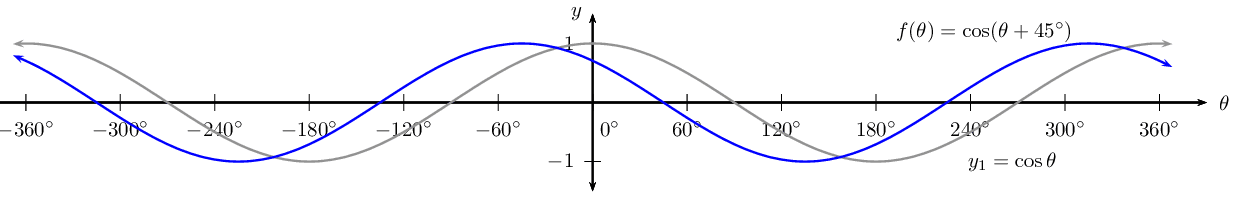$$g(\theta) =\cos (\theta - \text{30}\text{°})$$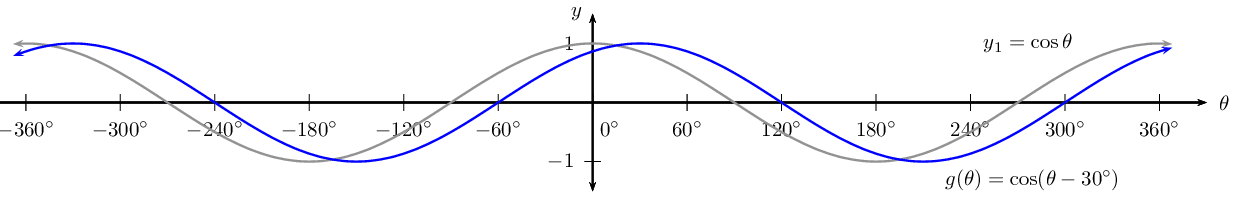$$h(\theta) =\cos (\theta + \text{60}\text{°})$$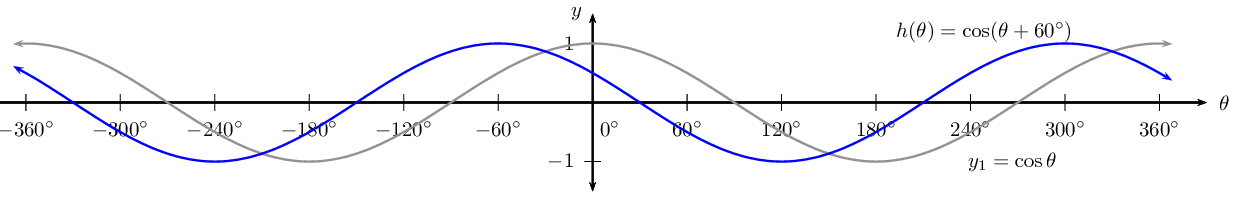## Worked example 24: Sketching a cosine graph

Sketch the graph of $$f(\theta) = \cos (\text{180}\text{°} - 3\theta)$$ for $$\text{0}\text{°} \leq \theta \leq \text{360}\text{°}$$.

### Examine the form of the equation

Write the equation in the form $$y = \cos k(\theta + p)$$. \begin{align*} f(\theta) &= \cos (\text{180}\text{°

To draw a graph of the above function, the standard cosine graph, $$y = \cos \theta$$, must be changed in the following ways:

• decrease the period by a factor of $$\text{3}$$
• shift to the right by $$\text{60}\text{°}$$.

### Complete a table of values

 θ $$\text{0}$$$$\text{°}$$ $$\text{45}$$$$\text{°}$$ $$\text{90}$$$$\text{°}$$ $$\text{135}$$$$\text{°}$$ $$\text{180}$$$$\text{°}$$ $$\text{225}$$$$\text{°}$$ $$\text{270}$$$$\text{°}$$ $$\text{315}$$$$\text{°}$$ $$\text{360}$$$$\text{°}$$ $$f(\theta)$$ $$-\text{1}$$ $$\text{0,71}$$ $$\text{0}$$ $$-\text{0,71}$$ $$\text{1}$$ $$-\text{0,71}$$ $$\text{0}$$ $$\text{0,71}$$ $$-\text{1}$$

### Plot the points and join with a smooth curve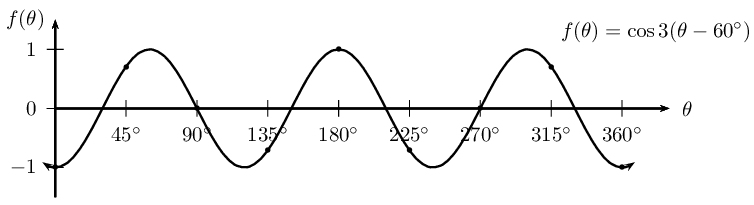Period: $$\text{120}$$$$\text{°}$$

Amplitude: $$\text{1}$$

Domain: $$[\text{0}\text{°};\text{360}\text{°}]$$

Range: $$[-1;1]$$

Maximum turning point: $$(\text{60}\text{°};1)$$, $$(\text{180}\text{°};1)$$ and $$(\text{300}\text{°};1)$$

Minimum turning point: $$(\text{0}\text{°}; -1)$$, $$(\text{120}\text{°};-1)$$, $$(\text{240}\text{°};-1)$$ and $$(\text{360}\text{°};-1)$$

$$y$$-intercepts: $$(\text{0}\text{°};-1)$$

$$x$$-intercept: $$(\text{30}\text{°};0)$$, $$(\text{90}\text{°};0)$$, $$(\text{150}\text{°};0)$$, $$(\text{210}\text{°};0)$$, $$(\text{270}\text{°};0)$$ and $$(\text{330}\text{°};0)$$

## Worked example 25: Finding the equation of a cosine graph

Given the graph of $$y = a \cos (k\theta + p)$$, determine the values of $$a$$, $$k$$, $$p$$ and the minimum turning point.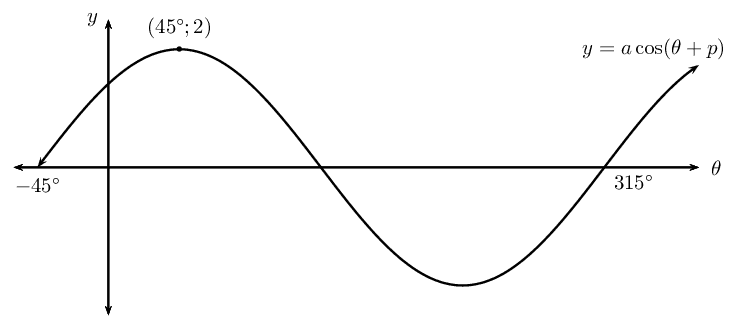### Determine the value of $$k$$

From the sketch we see that the period of the graph is $$\text{360}\text{°}$$, therefore $$k = 1$$.

$y = a \cos ( \theta + p)$

### Determine the value of $$a$$

From the sketch we see that the maximum turning point is $$(\text{45}\text{°};2)$$, so we know that the amplitude of the graph is $$\text{2}$$ and therefore $$a = 2$$.

$y = 2 \cos ( \theta + p)$

### Determine the value of $$p$$

Compare the given graph with the standard cosine function $$y = \cos \theta$$ and notice the difference in the maximum turning points. We see that the given function has been shifted to the right by $$\text{45}$$$$\text{°}$$, therefore $$p = \text{45}\text{°}$$.

$y = 2 \cos ( \theta - \text{45}\text{°})$

### Determine the minimum turning point

At the minimum turning point, $$y = -2$$:

\begin{align*} y &= 2 \cos ( \theta - \text{45}\text{°}) \\ -2 &= 2 \cos ( \theta - \text{45}\text{°}) \\ -1 &= \cos ( \theta - \text{45}\text{°}) \\ \cos^{-1}(-1) &= \theta - \text{45}\text{°} \\ \text{180}\text{°} &= \theta - \text{45}\text{°} \\ \text{225}\text{°} &= \theta \end{align*}

This gives the point $$(\text{225}\text{°};-2)$$.

## The cosine function

Textbook Exercise 5.27

Sketch the following graphs on separate axes:

$$y = \cos (\theta + \text{15}\text{°})$$ for $$-\text{180}\text{°} \leq \theta \leq \text{180}\text{°}$$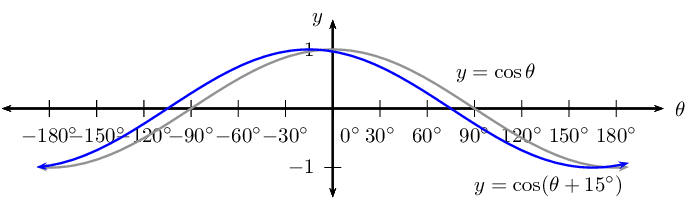$$f(\theta) = \frac{1}{3} \cos (\theta - \text{60}\text{°})$$ for $$-\text{90}\text{°} \leq \theta \leq \text{90}\text{°}$$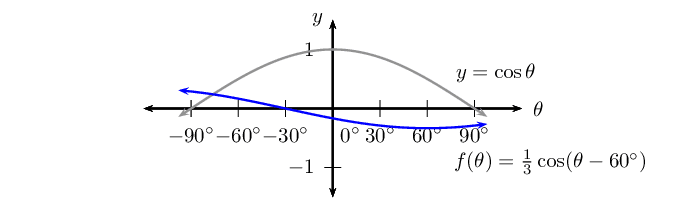$$y = -2 \cos \theta$$ for $$\text{0}\text{°} \leq \theta \leq \text{360}\text{°}$$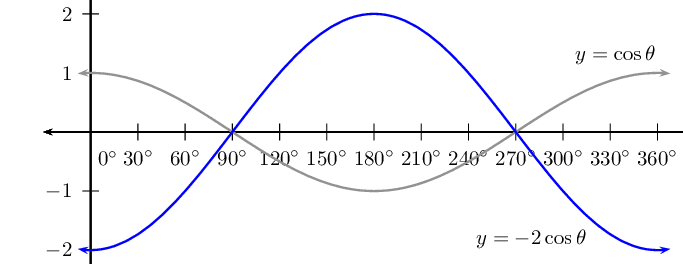$$y = \cos (\text{30}\text{°} - \theta)$$ for $$-\text{360}\text{°} \leq \theta \leq \text{360}\text{°}$$

\begin{align*} y &= \cos (\text{30}\text{°} - \theta) \\ &= \cos \left( -(\theta - \text{30}\text{°}) \right) \\ &= \cos \left(\theta - \text{30}\text{°} \right) \end{align*}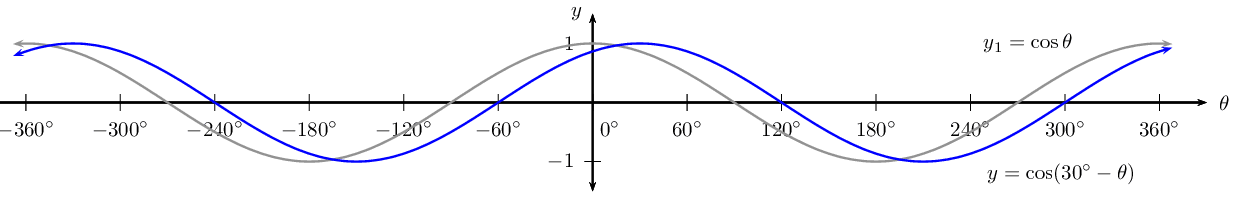$$g(\theta) = 1 + \cos (\theta - \text{90}\text{°})$$ for $$\text{0}\text{°} \leq \theta \leq \text{360}\text{°}$$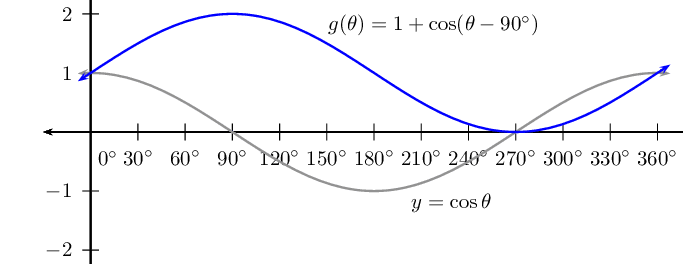$$y = \cos (2 \theta + \text{60}\text{°})$$ for $$-\text{360}\text{°} \leq \theta \leq \text{360}\text{°}$$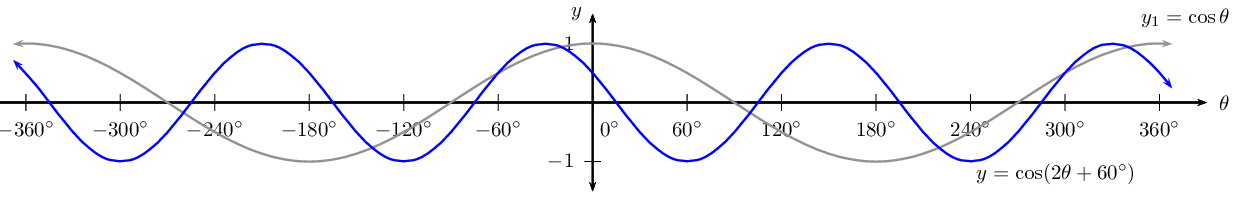Two girls are given the following graph: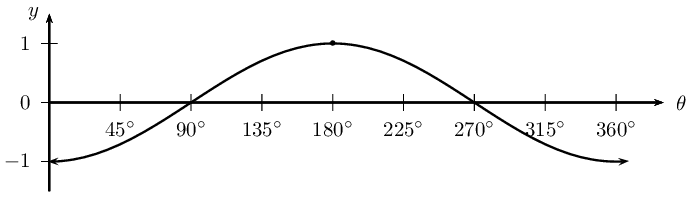Audrey decides that the equation for the graph is a cosine function of the form $$y = a \cos \theta$$. Determine the value of $$a$$.

$$a = -1$$

Megan thinks that the equation for the graph is a cosine function of the form $$y = \cos (\theta + p)$$. Determine the value of $$p$$.

$$p = -\text{180}\text{°}$$

What can they conclude?

$$\cos (\theta - \text{180}\text{°}) = -\cos \theta$$